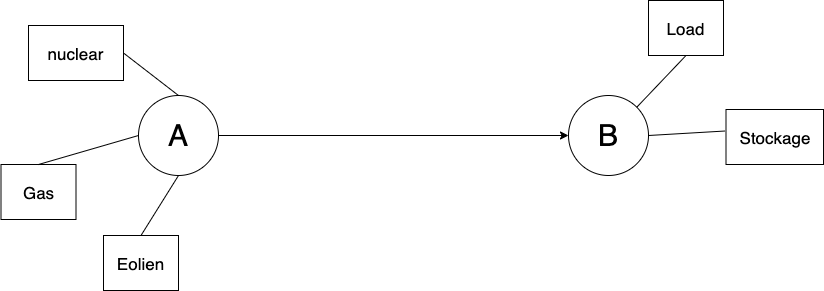# Storage¶

Except where otherwise noted, this content is Copyright (c) 2020, RTE and licensed under a CC-BY-4.0 license.

We has already seen Consumption, Production and Link to attach on node. Hadar has also a Stockage element. We will work on a simple network with two nodes : one with two producitons (stochastic and constant) other with consumption and stockageimg

import numpy as np


## Create data¶

np.random.seed(12684681)
eolien = np.random.rand(168) * 500 + 200 # random from 200 to 700
load = np.sin(np.linspace(-1, -1+np.pi*14, 168)) * 250 + 750 # sinus moving 500 to 1000


Start storage by remove storage !

study = hd.Study(horizon=eolien.size)\
.network()\
.node('a')\
.production(name='gas', cost=100, quantity=200)\
.production(name='nulcear', cost=50, quantity=300)\
.production(name='eolien', cost=10, quantity=eolien)\
.node('b')\

plot_without.network().node('b').stack()